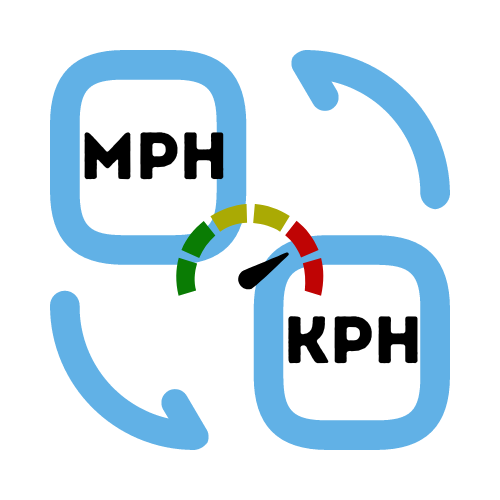# MPH to KPH Calculator

## Convert between miles per hour and kilometers per hour

• Created by Emily Rodriguez
• Reviewed by Ramesh Agarwal

## What is the difference between KM and MPH?

Kilometers (KM) is a measure of distance. Kilometer is also a way of measuring speed, where normally it is measured against time such as kilometers per hour (km/h or kph).

MPH means miles per hour, another measurement of speed. Sometimes you may also see it written as KMH, which also has the same meaning. Both miles per hour (mph) and kilometers per hour (kph) are common measurements of speed for road vehicles such as cars, trucks and motorbikes.

To encourage road safety, speed limits are assigned to roads and highways to reduce the casualties caused by driving at high speeds.

## How to convert between km/h and mph?First we need to find the difference between the measurements of 1 mile and 1 kilometer. When comparing distances, `1 mile = 1.60934 kilometer`.

To convert miles per hour to kilometers per hour (mph to kmh), we need to multiply the miles per hour value by 1.60934 to get the result in kilometers per hour.

We can also reverse this to convert kilometers per hour to miles per hour. This time we will need to divide the amount in kilometers by 1.60934 to get the result in miles per hour. As you can see, it's easy to switch between km/h and mph as needed.

## Example Conversion of KMH and MPH

Converting miles per hour (MPH) to kilometers per hour (km/h) is a simple division problem. If a car is going 60 miles per hour (mph) then you can do the following:

`60 x 1.60934 = 96.56 kph`

The result of this conversion is 96.56 km/h (or KPH), which we can then round to 97 kilometers per hour. If you want to see more conversions like this then check out the MPH to KM Conversion Chart at the bottom of this page.

## MPH to KM Conversion Chart

If you need to convert speed between miles per hour and kilometers per hour then the mph to kph chart and the kph to mph chart should make converting between these values a breeze. We've added the most common speeds in the tables below to save you time.

### MPH to KPH Chart

This miles per hour to kilometers per hour conversion table contains frequently used speeds for easy calculations. Find the value in MPH (left column) and the result in KPH or KMH (right column).

Miles per hour Kilometers per hour
10 MPH 16 KPH
20 MPH 32 KPH
30 MPH 48 KPH
40 MPH 64 KPH
50 MPH 80 KPH
60 MPH 97 KPH
70 MPH 113 KPH
80 MPH 129 KPH
90 MPH 145 KPH
100 MPH 161 KPH
110 MPH 177 KPH
120 MPH 193 KPH
130 MPH 209 KPH
140 MPH 225 KPH
150 MPH 241 KPH

### KPH to MPH Chart

This kilometers per hour to miles per hour conversion table contains frequently used speeds for quick and easy conversions. Find the speed in KPH (left column) and the result in MPH (right column).

Kilometers per hour Miles per hour
10 KPH 6 MPH
20 KPH 12 MPH
30 KPH 19 MPH
40 KPH 25 MPH
50 KPH 31 MPH
60 KPH 37 MPH
70 KPH 43 MPH
80 KPH 50 MPH
90 KPH 56 MPH
100 KPH 62 MPH
110 KPH 68 MPH
120 KPH 75 MPH
130 KPH 81 MPH
140 KPH 87 MPH
150 KPH 93 MPH